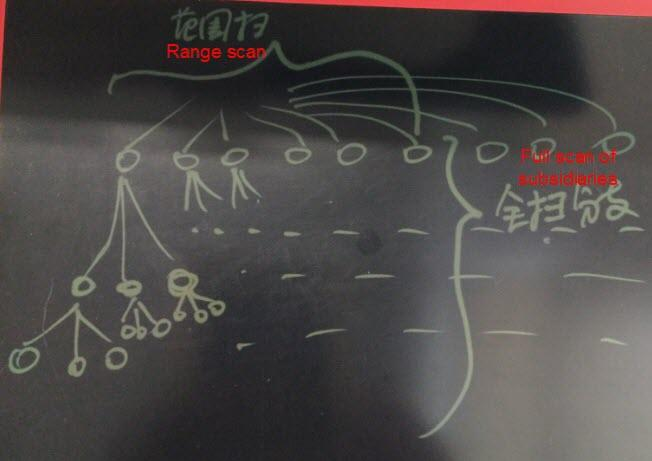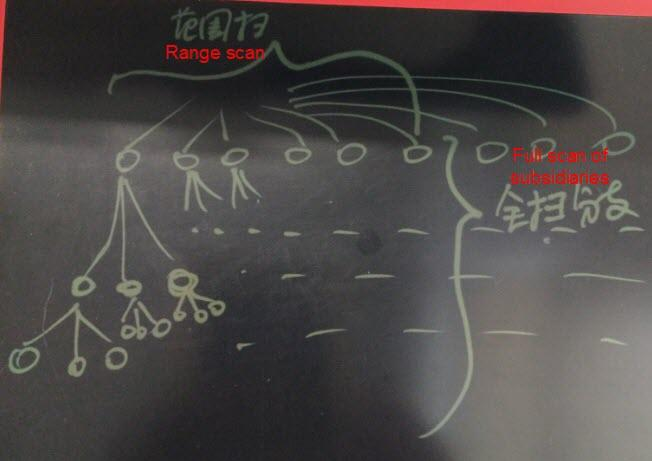# Community

Blog Events Webinars Tutorials Forum
×
Community Blog Accelerate Spatial Search Using Spatial Composite Indexing

# Accelerate Spatial Search Using Spatial Composite Indexing

In this article, the author discusses performance issues in spatial search and accelerating spatial search using GiST and B-tree spatial composite indexes.

By Digoal

## Background

With the mobile internet’s popularization, spatial data has become a standard configuration of most enterprises in the travel and express delivery industries.

Data queries are usually accompanied by location distance search and filtering by other attributes, such as time range, region ID, or a logistics company ID.

The spatial index and B-tree index are two types of indexes in PostgreSQL (PostgreSQL supports multiple indexing methods, such as B-tree, Hash, GIN, GiST, SP-GiST, BRIN, RUM, Bloom, and ZoomDB).

How to achieve optimal query efficiency?

## GiST Spatial Composite Index

Example:

Three key fields are stored in the database: one indicates the company of a shared bicycle, one indicates whether the shared bicycle is in use, and another indicates the current location of the shared bicycle.

Construct a test table containing three fields: two INT type fields and one POINT type field. Perform nearest neighbor queries on the data by point and filter out certain values of c1 and c2.

The test table and the test data are as follows:

``````postgres=# create table cb(
c1 int,  -- 0表示未使用，1表示已使用
c2 int,  -- 共享单车属于哪家运营公司
c3 point  -- 共享单车当前位置
);
CREATE TABLE

postgres=# insert into cb select random()*1, random()*1000 , point(10000*random(), 10000*random()) from generate_series(1,10000000);
INSERT 0 10000000

postgres=# select * from cb limit 10;
c1 | c2  |                 c3
----+-----+-------------------------------------
0 | 981 | (8099.59028847516,9043.13919134438)
1 | 256 | (9331.68333489448,2223.74511882663)
1 | 510 | (2517.2486435622,8716.1894608289)
0 | 398 | (2658.8175073266,2361.14453990012)
0 | 989 | (8130.69586176425,1361.2649217248)
0 | 344 | (2282.57383685559,9480.9684343636)
1 | 944 | (8550.47187302262,2814.43384941667)
0 | 418 | (3858.46449527889,5060.3136094287)
0 | 196 | (4103.45280077308,1458.2177111879)
0 | 344 | (3681.96283001453,1260.5628464371)
(10 rows)  ``````

Search for idle shared bicycles of a specific company within a distance of 1000 meters away from a certain point.

The query statement is as follows:

``select * from cb where c1=0 and c2=100 and c3 <@ circle '((23,3175),1000)' order by c3 <-> point(23,3175) limit 1000;  ``

Create a spatial composite index.

``````postgres=# set maintenance_work_mem='32GB';
SET

postgres=# create extension btree_gist;
CREATE EXTENSION

postgres=# create index idx1 on cb using gist(c1,c2,c3);
CREATE INDEX  ``````

The performance is as follows:

``````postgres=# explain (analyze,verbose,timing,costs,buffers) select * from cb where c1=0 and c2=100 and c3 <@ circle '((23,3175),1000)' order by c3 <-> point(23,3175) limit 1000;
QUERY PLAN
-----------------------------------------------------------------------------------------------------------------------------
Limit  (cost=0.42..9.55 rows=5 width=32) (actual time=0.125..0.355 rows=93 loops=1)
Output: c1, c2, c3, ((c3 <-> '(23,3175)'::point))
Buffers: shared hit=106
->  Index Only Scan using idx1 on public.cb  (cost=0.42..9.55 rows=5 width=32) (actual time=0.124..0.344 rows=93 loops=1)
Output: c1, c2, c3, (c3 <-> '(23,3175)'::point)
Index Cond: ((cb.c1 = 0) AND (cb.c2 = 100) AND (cb.c3 <@ '<(23,3175),1000>'::circle))
Order By: (cb.c3 <-> '(23,3175)'::point)
Heap Fetches: 93
Buffers: shared hit=106
Planning time: 0.110 ms
Execution time: 0.387 ms
(11 rows)  ``````

### Example with PostGIS

For the following PostGIS-related query:

``````explain (analyze,verbose,timing,costs,buffers)
select xxx1,xxx2,xxx3,st_asbinary(geo) as geo,
ST_Transform (ST_GeomFromText ('POINT(121.403833486783 31.1425794813889)', 4326), 26986) <-> ST_Transform (geo, 26986) as distance2Center
from tbl
where xxx1='1' and xxx2='xxx'
and ST_Transform (geo, 26986) && ST_Buffer(ST_Transform(ST_GeomFromText('POINT(121.403833486783 31.1425794813889)', 4326), 26986), 300)
order by ST_Transform (ST_GeomFromText ('POINT(121.403833486783 31.1425794813889)', 4326), 26986) <-> ST_Transform (geo, 26986) asc

create index idx1 on tbl using gist(xxx1, xxx2, ST_Transform (geo, 26986));``````

The query optimization is as follows:

``````create or replace function ff1(geometry, float8, int) returns setof record as \$\$
declare
v_rec record;
v_limit int := \$3;
begin
set local enable_seqscan=off;   -- 强制索引, 扫描行数够就退出.
for v_rec in
select *,
ST_Distance ( \$1, loc_box ) as dist
from cloudpoint_test_agg
-- where xxx1='1' and xxx2='xxx'
order by loc_box <-> \$1           -- 按距离顺序由近到远返回
loop
if v_limit <=0 then               -- 判断返回的记录数是否达到LIMIT的记录数
raise notice '已经取足limit设置的 % 条数据, 但是距离 % 以内的点可能还有.', \$3, \$2;
return;
end if;
if v_rec.dist > \$2 then       -- 判断距离是否大于请求的距离
raise notice '距离 % 以内的点已输出完毕', \$2;
return;
else
return next v_rec;
end if;
v_limit := v_limit - array_length(v_rec.loc_agg, 1);  -- 扣减grid内的point个数
end loop;
end;
\$\$ language plpgsql strict volatile; ``````

### Performance Deteriorates Greatly Without the Spatial Composite Index

For the following data (same as the data above):

``````postgres=# create table cc (like cb);
CREATE TABLE
postgres=# insert into cc select * from cb;
INSERT 0 10000000

postgres=# create index idx2 on cc using gist(c3);
CREATE INDEX  ``````

The query performance is as follows:

``````postgres=# explain (analyze,verbose,timing,costs,buffers) select * from cc where c1=0 and c2=100 and c3 <@ circle '((23,3175),1000)' order by c3 <-> point(23,3175) limit 1000;
QUERY PLAN
--------------------------------------------------------------------------------------------------------------------------------------
Limit  (cost=12552.41..12552.42 rows=5 width=32) (actual time=153.300..153.317 rows=93 loops=1)
Output: c1, c2, c3, ((c3 <-> '(23,3175)'::point))
Buffers: shared hit=60543
->  Sort  (cost=12552.41..12552.42 rows=5 width=32) (actual time=153.298..153.306 rows=93 loops=1)
Output: c1, c2, c3, ((c3 <-> '(23,3175)'::point))
Sort Key: ((cc.c3 <-> '(23,3175)'::point))
Sort Method: quicksort  Memory: 32kB
Buffers: shared hit=60543
->  Bitmap Heap Scan on public.cc  (cost=236.92..12552.35 rows=5 width=32) (actual time=52.341..153.244 rows=93 loops=1)
Output: c1, c2, c3, (c3 <-> '(23,3175)'::point)
Recheck Cond: (cc.c3 <@ '<(23,3175),1000>'::circle)
Filter: ((cc.c1 = 0) AND (cc.c2 = 100))
Rows Removed by Filter: 160633
Heap Blocks: exact=58622
Buffers: shared hit=60543
->  Bitmap Index Scan on idx2  (cost=0.00..236.92 rows=10000 width=0) (actual time=39.223..39.223 rows=160726 loops=1)
Index Cond: (cc.c3 <@ '<(23,3175),1000>'::circle)
Buffers: shared hit=1921
Planning time: 0.116 ms
Execution time: 153.373 ms
(20 rows)

postgres=# set enable_seqscan=off;
SET
postgres=# explain (analyze,verbose,timing,costs,buffers) select * from cc where c1=0 and c2=100 and c3 <@ circle '((23,3175),1000)' order by c3 <-> point(23,3175) limit 1000;
QUERY PLAN
------------------------------------------------------------------------------------------------------------------------------
Limit  (cost=0.42..14296.43 rows=5 width=32) (actual time=0.998..210.033 rows=93 loops=1)
Output: c1, c2, c3, ((c3 <-> '(23,3175)'::point))
Buffers: shared hit=162645
->  Index Scan using idx2 on public.cc  (cost=0.42..14296.43 rows=5 width=32) (actual time=0.996..210.008 rows=93 loops=1)
Output: c1, c2, c3, (c3 <-> '(23,3175)'::point)
Index Cond: (cc.c3 <@ '<(23,3175),1000>'::circle)
Order By: (cc.c3 <-> '(23,3175)'::point)
Filter: ((cc.c1 = 0) AND (cc.c2 = 100))
Rows Removed by Filter: 160633
Buffers: shared hit=162645
Planning time: 0.109 ms
Execution time: 210.079 ms
(12 rows)  ``````

The poor performance is caused by rows removed by a filter. Many unnecessary operations are performed because many rows do not meet the filter conditions during filter by space scanning.

## B-tree Composite Index (Geohash + Other Filtering Conditions)

If you use the geohash type rather than the geometry type and your geographic location is not a boundary address, adjacent data may have the same geohash prefix. Therefore, use a B-tree index for geohashing.

``````create table test (
c1 int, -- 共享单车是否已被租用
c2 int, -- 共享单车运营公司
c3 text  -- 共享单车位置(geohash)
);

create index idx on test using btree(c1,c2,c3);  ``````

Further, optimize search performance by clustering to reduce the discrete degree of index scanning.

``cluster test using idx;  ``

## Composite Index Optimization for Range Scan

Here is the reason why the performance in the previous example is so low when the filter condition in the driver column is a range rather than "equal to":

The entire range and its subsidiaries need to be scanned, but the index block is a discrete block, resulting in low scan efficiency.Example:

``````create table test(c1 int, c2 int, c3 timestamp, c4 point);

create index idx on test using gist(c3,c2,c4);

select * from test where c3 between '2017-01-01' and '2017-01-02' and c2=1 order by c4;

In this example, the points in the driver column are scanned, resulting in high efficiency.How to deal with scenarios with range scan?

Use a partitioned table. For example, use the C3 field in the partition column to partition a table by time. Remove the C3 column when creating an index.

``````create table test_20170101 (like test, check c3 between '2017-01-01' and '2017-01-02');

create index idx on test_20170101 using gist (c2, c4);

select * from test_20170101 where c2=1 order by c4;  ``````

Alternatively, optimize the kernel to enable it to support partition indexing.

### Kernel Optimization

Partition indexes by time to create partitioned indexes.

During a scan, specified index partitions are retrieved by default. This achieves the same effect as using a partition table and independent indexes.

### Suggestions from Experts in the GIS Field

We use PostgreSQL's built-in geometric point data types instead of GIS data types in this article to facilitate testing. Please take the following suggestions from experts in the GIS field from the PostgreSQL community to avoid confusion.

``````select DropGeometryColumn ('cb','geom');
drop table if exists  cb;

create table cb(
objectid int not null, --共享单车编号
c1 int,                -- 0表示未使用，其它表示已使用
c2 int,                -- 共享单车属于哪家运营公司
constraint pk_cb_objectid primary key (objectid)
);

--GIS必须明确指出地图单位是什么

--民用GPS小数6位精度(6位大约为10米级精度,8位已经是亚米级精度)已经很高了,再多没有意义

--c3 point  -- 共享单车当前位置, point在文章里只能算是自定义类型(实际上是PostgreSQL内置的几何点类型),这会给参考您文章的学习GIS的同学造成困扰

--where c1=0 and c2=100 and c3 <@ circle '((23,3175),1000)' order by c3 <-> point(23,3175) limit 1000;

--搜索某个点附近1000距离内，属于某个公司的，没有使用的共享单车。

--这样的查询条件在测试没问题,但是别人看了会造成困扰，因为没有地图单位,这个1000距离不知道是什么东西

--新版本的postgis文档中已经没有ST_GeomFromText ('POINT(121.403833486783 31.1425794813889)', 4326)这样的函数了,虽然还支持,未来可能会删除

--请使用ST_SetSRID(ST_GeomFromText ('POINT(121.403833486783 31.1425794813889)'),4326)或ST_GeomFromText ('SRID=4326;POINT(121.403833486783 31.1425794813889)')

--发现好几篇您写的关于gis的文章都有类似的问题,希望关于gis方面的文章严格按postgis标准

--创建空间字段,根据空间参考不同,地图单位可能为米、度或其它，统称地图单位

select AddGeometryColumn ('cb','geom',4326,'POINT',2); -- 共享单车当前位置,GPS采用4326 ,类型为点,二维坐标

--创建空间索引
create index gidx_cb_geom on cb using gist(geom);
create index gidx_cb_geomgraphy on cb using gist((geom::geography));

--坐标范围限制在中国[73.406586, 3.408477, 135.085831, 53.880950]
do \$\$
declare vStart bigint;
declare vEnd bigint;
declare MAXVALE bigint;
declare INTERVAL bigint;
begin
MAXVALE := 20000000;
INTERVAL := 1000; vStart := 1 ;vEnd := INTERVAL;
loop
-- 20家公司比较符合市场现状,更能反应实际情况
insert into cb
select id,(random()*1)::integer, (random()*(20-1)+1)::integer,
ST_SetSRID(ST_Point(
round((random()*(135.085831-73.406586)+73.406586)::numeric,6),
round((random()*(53.880950-3.408477)+3.408477)::numeric,6)
),4326)
from generate_series(vStart,vEnd) as id;
raise notice  '%', vEnd;
vStart := vEnd + 1; vEnd := vEnd + INTERVAL;
if( vEnd > MAXVALE ) then
return;
end if;
end loop;
end\$\$;

--ix,iy为gps经度和纬度(单位为度)

--idistance为搜索距离(单位为米)

drop function if exists spatialQuery(ix float,iy float,idistance float);
create or replace function spatialQuery(ix float,iy float,idistance float)
returns table(oobjectid integer,oc1 integer,oc2 integer,odistance float,ogeom geometry)
as \$\$
declare
vrecord record;
vcurrentpoint geometry;
vspheroid  spheroid;
begin
vspheroid := 'SPHEROID["WGS84",6378137,298.257223563]' ;  --WGS84椭球体参数定义
vcurrentpoint := ST_SetSRID(ST_Point(ix,iy),4326);  --
--查找圆心为vcurrentpoint,半径idistance米范围内未使用的共享单车,并按距离排序,只返回1千行
return query     ( with cte as(
select * from cb
where ST_DWithin(geom::geography ,vcurrentpoint::geography,idistance,true)
) select objectid,c1,c2,ST_DistanceSpheroid(geom,vcurrentpoint,vspheroid),geom
from cte where c1=0 order by ST_DistanceSpheroid(geom,vcurrentpoint,vspheroid)  limit 1000 );
end;
\$\$ language plpgsql;

select * from spatialQuery(102,24,5000);

--查询计划

explain (analyze,verbose,costs,buffers,timing)
with cte as(
select * from cb
where ST_DWithin(geom::geography ,ST_SetSRID(ST_Point(102,24),4326)::geography,5000,true)
) select objectid,c1,c2,ST_DistanceSpheroid(geom,ST_SetSRID(ST_Point(102,24),4326),'SPHEROID["WGS84",6378137,298.257223563]'),geom
from cte where c1=0 order by ST_DistanceSpheroid(geom,ST_SetSRID(ST_Point(102,24),4326),'SPHEROID["WGS84",6378137,298.257223563]')  limit 1000;``````

## Summary

1) If you want to achieve the optimal query performance with a composite index, we recommend using equivalent queries instead of range queries in the driver column because a range query causes a broader range search.

2) If you use a composite index for sorting, we recommend performing the sorting either by all fields or by the equivalence condition in the driver column and the suffix column.

3) To reduce the discrete degree of index scans, we recommend that you cluster data by index.

0 0 0
Share on

# Related Products

• ##Database Overview

Fully managed and less trouble database services

• ##AnalyticDB for PostgreSQL

An online MPP warehousing service based on the Greenplum Database open source program

• ##ApsaraDB RDS for PostgreSQL

An on-demand database hosting service for PostgreSQL with automated monitoring, backup and disaster recovery capabilities

• ##Database for FinTech Solution

Leverage cloud-native database solutions dedicated for FinTech.